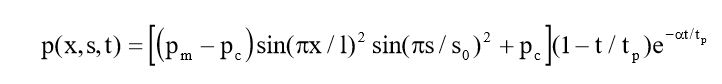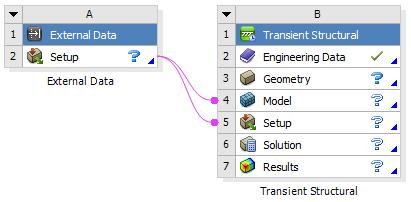# Time Dependent Non-Uniform Pressure Function

Hello everyone,

I have an assignment which have time dependent and non-uniform pressure function. But i could not find how to add and analyze this function. Can anybody know how i can do it ? (Btw i am not sure about the category, if it is wrong please let me know)

• edited June 30

It might help if you tell us which solver you're using. In Fluent you want UDFs, profiles or expressions, in CFX it's expressions or user routines, Mechanical will be different again.

• I am trying to use mechanical.We can think x and s as x and y actually. I could not find a way to insert this function.

• Have you implemented this in Excel or matlab?

Create a grid of 11 points in x and 11 points in y that span an area from (0,0) to (l,s0) that compute pressure values on a time increment that is some fraction of tp such as tp/100.  It may be useful to have those 12100 pressure values in a table of x,y,t for use in Mechanical which can import the table into a model using the External Data component in Workbench.

This will only be useful if the mechanical model has the same geometric dimension of (l,s0) and is rectangular.If you want finer detail, you can use more than 11 points in each direction and more than 100 points in time, I'm just giving you a concrete example.

• Thank you Peter. But unfortunately my mechanical model is not rectangular.